﻿ 二维圆盘中不同外场对等离激元激发的影响 Effect of the Different External Fields on Plasmon Excitations in Two-Dimensional Disk

Applied Physics
Vol. 08  No. 09 ( 2018 ), Article ID: 26745 , 8 pages
10.12677/APP.2018.89052

Effect of the Different External Fields on Plasmon Excitations in Two-Dimensional Disk

Hongjie Xue*, Huachun Wu, Zhi Yao, Xiaomei Wang

School of Science, Xi’an Aeronautical University, Xi’an Shannxi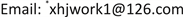Received: Aug. 19th, 2018; accepted: Sep. 3rd, 2018; published: Sep. 10th, 2018ABSTRACT

We study the plasmon excitations in two-dimensional disk, based on the linear response theory in the random-phase approximation and the free-electron gas model. With the help of energy absorption spectrum and charge distribution, the effect of external electric fields on plasmon is obtained. Results show that the uniform external electric field only can excite quadrupole plasmon; the non-uniform external electric field can excite both quadrupole plasmon and quadrupole plasmon.

Keywords:Two-Dimensional Disk, Plasmon, The Linear Response Theory, The Free-Electron Gas Mode1. 引言

2. 研究方法

${\rho }^{ind}\left(r,\omega \right)=\int \Pi \left(r,{r}^{\prime },\omega \right)V\left({r}^{\prime },\omega \right)\text{d}{r}^{\prime }$ (1)

$\int \Pi \left(r,{r}^{\prime },\omega \right)=2{e}^{2}\sum _{nm}\frac{f\left({E}_{n}\right)-f\left({E}_{m}\right)}{{E}_{n}-{E}_{m}-\omega -i\gamma }{\psi }_{n}^{\ast }\left(r\right){\psi }_{m}\left(r\right){\psi }_{m}^{\ast }\left({r}^{\prime }\right){\psi }_{n}\left({r}^{\prime }\right)$ (2)

${V}^{ind}\left(r,\omega \right)=\frac{1}{4\text{π}{\epsilon }_{0}}\int \frac{{\rho }^{ind}\left({r}^{\prime },\omega \right)}{|r-{r}^{\prime }|}\text{d}{r}^{\prime }$ (3)

${V}^{ind}\left(r,\omega \right)=2{e}^{2}\sum _{nm}\frac{f\left({E}_{n}\right)-f\left({E}_{m}\right)}{{E}_{n}-{E}_{m}-\omega -i\gamma }×\frac{1}{4\text{π}{\epsilon }_{0}}\int \frac{{\psi }_{n}^{\ast }\left({r}^{\prime }\right){\psi }_{m}\left({r}^{\prime }\right)}{|r-{r}^{\prime }|}\text{d}{r}^{\prime }\left[{V}_{nm}^{ext}\left(\omega \right)+{V}_{nm}^{ind}\left(\omega \right)\right]$ (4)

${V}_{nm}\left(\omega \right)=\int {\psi }_{n}^{\ast }\left(r\right)V\left(r,\omega \right){\psi }_{m}\left(r\right)$ (5)

${V}_{{n}^{\prime }{m}^{\prime }}^{ind}\left(\omega \right)=2{e}^{2}\sum _{nm}\frac{f\left({E}_{n}\right)-f\left({E}_{m}\right)}{{E}_{n}-{E}_{m}-\omega -i\gamma }×\frac{1}{4\text{π}{\epsilon }_{0}}\int \frac{{\psi }_{n}^{\ast }\left({r}^{\prime }\right){\psi }_{m}\left({r}^{\prime }\right){\psi }_{{n}^{\prime }}^{\ast }\left(r\right){\psi }_{{m}^{\prime }}\left(r\right)}{|r-{r}^{\prime }|}\left[{V}_{nm}^{ext}\left(\omega \right)+{V}_{nm}^{ind}\left(\omega \right)\right]\text{d}{r}^{\prime }\text{d}r$ (6)

${M}_{{n}^{\prime }{m}^{\prime },nm}\left(\omega \right)=\frac{2{e}^{2}}{4\text{π}{\epsilon }_{0}}\sum _{nm}\frac{f\left({E}_{n}\right)-f\left({E}_{m}\right)}{{E}_{n}-{E}_{m}-\omega -i\gamma }\int \frac{{\psi }_{{n}^{\prime }}^{\ast }\left(r\right){\psi }_{{m}^{\prime }}\left(r\right){\psi }_{n}^{\ast }\left({r}^{\prime }\right){\psi }_{m}\left({r}^{\prime }\right)}{|r-{r}^{\prime }|}\text{d}{r}^{\prime }\text{d}r$ (7)

$\sum _{nm}\left[{\delta }_{{n}^{\prime }{m}^{\prime },nm}-{M}_{{n}^{\prime }{m}^{\prime },nm}\left(\omega \right)\right]{V}_{nm}^{ind}\left(\omega \right)=\sum _{nm}{M}_{{n}^{\prime }{m}^{\prime },nm}\left(\omega \right){V}_{nm}^{ext}\left(\omega \right)$ (8)

$A\left(\omega \right)=-\frac{1}{2}\omega \mathrm{Im}\left\{\int {\rho }^{ind}\left(r,\omega \right){\left[{V}^{ext}\left(r,\omega \right)\right]}^{\ast }\right\}$ (9)

${\psi }_{nk}\left(r,\theta \right)=\sqrt{\frac{1}{\text{π}{R}_{\text{0}}^{\text{2}}}}\frac{{J}_{n}\left(\frac{{x}_{nk}}{{R}_{\text{0}}^{\text{2}}}r\right)}{{J}_{n-1}\left({x}_{nk}\right)}{e}^{±in\theta }$ (10)

${E}_{nk}=\frac{{\hslash }^{2}{x}_{nk}^{2}}{2{m}_{e}{R}_{\text{0}}^{\text{2}}}$ (11)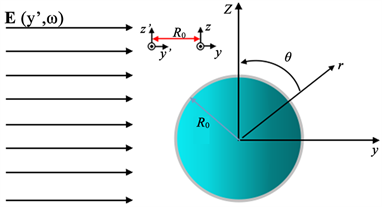Figure 1. The two-dimensional disk model in the external electric field incident

${\psi }_{nk}\left(r,\theta \right)=C{R}_{n}\left({N}_{k}r\right){e}^{±in\theta }$ (12)

${\rho }^{ind}\left(r,\theta ,\omega \right)=\sum _{m}{\rho }_{m}^{ind}\left(r,\omega \right){e}^{im\theta }$ (13)

${V}^{ind}\left(r,\theta ,\omega \right)=\sum _{m}{V}_{m}^{ind}\left(r,\omega \right){e}^{im\theta }$ (14)

$\begin{array}{c}{\rho }_{m}^{ind}\left(r,\omega \right)=4\text{π}{e}^{2}\left[\sum _{nk,{k}^{\prime }}\left(\frac{1}{{E}_{nk}-{E}_{\left(n+m\right){k}^{\prime }}-\omega -i\gamma }+\frac{1}{{E}_{nk}-{E}_{\left(n+m\right){k}^{\prime }}+\omega +i\gamma }\right)\right]\\ ×{C}^{2}{R}_{n}\left({N}_{k}r\right){R}_{n+m}\left({N}_{{k}^{\prime }}r\right)\left[{V}_{nk{k}^{\prime }}^{ext}\left(\omega \right)+{V}_{nk{k}^{\prime }}^{ind}\left(\omega \right)\right]\end{array}$ (15)

${V}_{m}^{ind}\left(r,\omega \right)=\int \frac{{r}^{\prime }{\rho }_{m}^{ind}\left({r}^{\prime },\omega \right){\text{e}}^{im\left(\theta -{\theta }^{\prime }\right)}}{4\text{π}{\epsilon }_{0}\sqrt{{r}^{2}+{{r}^{\prime }}^{2}-2r{r}^{\prime }\mathrm{cos}\left(\theta -{\theta }^{\prime }\right)}}\text{d}{r}^{\prime }\text{d}\left(\theta -{\theta }^{\prime }\right)$ (16)

${V}_{nk{k}^{\prime }}\left(\omega \right)={C}^{2}\int {r}^{\prime }{R}_{n+m}\left({N}_{{k}^{\prime }}{r}^{\prime }\right){R}_{n}\left({N}_{k}{r}^{\prime }\right){V}_{m}\left({r}^{\prime },\omega \right)\text{d}{r}^{\prime }$ (17)

$\sum _{nk{k}^{\prime }}\left[{\delta }_{ab{b}^{\prime }}-{M}_{ab{b}^{\prime },nk{k}^{\prime }}\left(\omega \right)\right]{V}_{nk{k}^{\prime }}^{ind}\left(\omega \right)=\sum _{nk{k}^{\prime }}{M}_{ab{b}^{\prime },nk{k}^{\prime }}\left(\omega \right){V}_{nk{k}^{\prime }}^{ext}\left(\omega \right)$ (18)(19)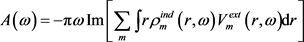(20)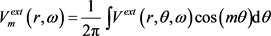(21)

3. 结果与分析Figure 2. Energy Absorption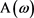with frequencyin the uniform external electric field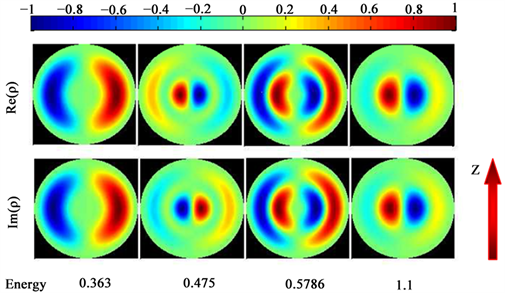Figure 3. The charge distribution of the plasmon excited by the uniform electric field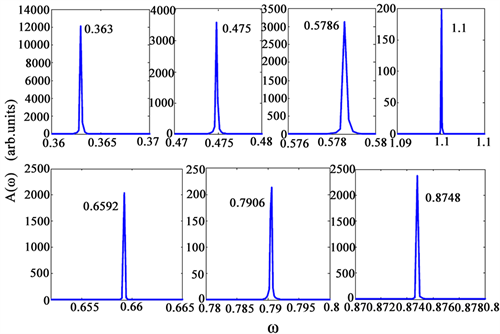Figure 4. Energy absorptionwith frequencyin the non-uniform electric field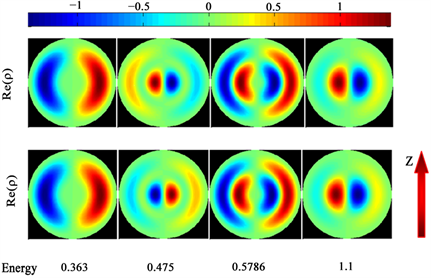Figure 5. The charge distribution of the plasmon excited by both the uniform field and the non-uniform field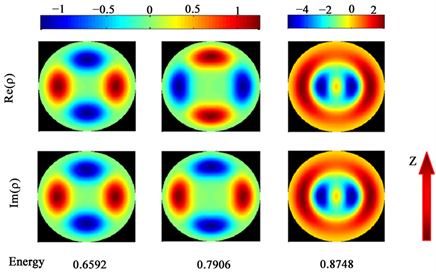Figure 6. The charge distribution of the plasmon excited only by the non-uniform field

4. 结论

Effect of the Different External Fields on Plasmon Excitations in Two-Dimensional Disk[J]. 应用物理, 2018, 08(09): 413-420. https://doi.org/10.12677/APP.2018.89052

1. 1. Huang, H., Wang, B. and Long, H. (2014) Plasmon-Negative Refraction at the Heterointerface of Graphene Sheet Arrays. Optics Letters, 39, 5957-5960. https://doi.org/10.1364/OL.39.005957

2. 2. 龚健, 张利伟, 陈亮. 石墨烯基双曲色散特异材料的负折射与体等离子体性[J]. 物理学报, 2015, 64(6): 67301-067301.

3. 3. Ghenuche, P., Cherukulappurath, S. and Taminiau, T.H (2008) Spectroscopic Mode Mapping of Resonant Plasmon Nanoantennas. Physical Review Letters, 101, 116805-116808. https://doi.org/10.1103/PhysRevLett.101.116805

4. 4. Kim, S., Jin, J. and Kim, Y. (2008) High-Harmonic Generation by Reso-nant Plasmon Field Enhancement. Nature, 453, 757-760. https://doi.org/10.1038/nature07012

5. 5. Mühlschlegel, P., Eisler, H.J. and Martin, O.J.F. (2005) Resonant Optical Antennas. Science, 308, 1607-1609. https://doi.org/10.1126/science.1111886

6. 6. 尹海峰, 毛力. 一维原子链局域等离激元的非线性激发[J]. 物理学报, 2016, 65(8): 321-327.

7. 7. Auguié, B. and Barnes, W.L. (2008) Collective Resonances in Gold Nanoparticle Arrays. Physical Review Letters, 101, 143902-143905. https://doi.org/10.1103/PhysRevLett.101.143902

8. 8. Hicks, E.M., Zou, S. and Schatz, G.C. (2005) Controlling Plasmon Line Shapes through Diffractive Coupling in Linear Arrays of Cylindrical Nanoparticles Fabricated by Electron Beam Lithography. Nano Letters, 5, 1065-1070. https://doi.org/10.1021/nl0505492

9. 9. Chu, Y., Schonbrun, E. and Yang, T. (2008) Experimental Observation of Narrow Sur-face Plasmon Resonances in Gold Nanoparticle Arrays. Applied Physics Letters, 93, 181108-181110. https://doi.org/10.1063/1.3012365

10. 10. Sun, Z., Jung, Y.S. and Kim, H.K. (2003) Role of Surface Plasmons in the Optical In-teraction in Metallic Gratings with Narrow Slits. Applied Physics Letters, 83, 3021-3023. https://doi.org/10.1063/1.1618021

11. 11. Barnes, W.L., Murray, W.A. and Dintinger, J. (2004) Surface Plasmon Polaritons and Their Role in the Enhanced Transmission of Light through Periodic Arrays of Subwavelength Holes in a Metal Film. Physical Review Letters, 92, 107401-107404. https://doi.org/10.1103/PhysRevLett.92.107401

12. 12. Wang, Q.J., Huang, C.P. and Li, J.Q. (2006) Suppression of Transmission Minima and Maxima with Structured Metal Surface. Applied Physics Letters, 89, 221121-221123. https://doi.org/10.1063/1.2400098

13. 13. Fang, X., Li, Z. and Long, Y. (2007) Surface-Plasmon-Polariton Assisted Diffraction in Periodic Subwavelength Holes of Metal Films with Reduced Interplane Coupling. Physical Review Letters, 99, 066805-066808. https://doi.org/10.1103/PhysRevLett.99.066805

14. 14. Xue, H.J., Hao, D.P, Zhang, M. and Wang, X.M. (2017) Plasmon Excita-tions in the Dimers Formed by Atom Chains. Physica E, 86, 292-296. https://doi.org/10.1016/j.physe.2016.10.030

15. 15. Xue, H.J., Wu, R.L. and Hu, C.X. (2018) The Study of the Plasmon Modes of Square Atomic Clusters Based on the Eigen-Oscillation Equation of Charge under the Free-Electron Gas Model. International Journal of Modern Physics B, 32, 1850139-1850148. https://doi.org/10.1142/S0217979218501394

16. NOTES

*通讯作者。